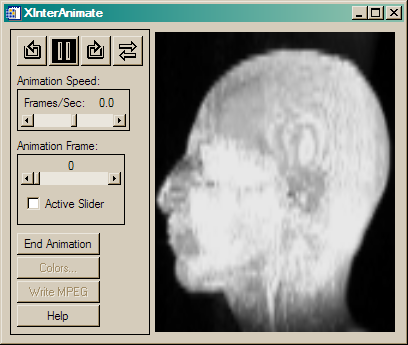# Maximum Intensity Projection of Image

QUESTION: I have a cube of image data (from an MRI scanner), and I would like to produce a maximum intensity image of the data. Is there an easy way to do that in IDL?ANSWER: Yes, you can do this quite easily using the DIMENSION keyword of either MAX (for a maximum intensity image) or MIN (for a minimum intensity image). Suppose, for example, you were using the MRI dataset of the head that is available in the IDL distribution:

```   head = BytArr(80,100,57)
file = Filepath(Subdirectory=['examples','data'], 'head.dat')
OpenR, lun, file, /Get_Lun
ReadU, lun, head
Free_Lun, lun
head = Rebin(head, 160, 200, 57)
```

To display a maximum intensity projection, you can type this:

```   IDL> TV, MAX(head, DIMENSION=3)
```

To display a minumum intensity projection, you can type this:

```   IDL> TV, MIN(head, DIMENSION=3)
```

If you wanted a display over some other dimension than the last one, you will have to use the REFORM command to eliminate the single dimension before you display the image. This is done automatically for you if the single dimension is the last dimension of an array.

```   IDL> TV, REFORM(MAX(head, DIMENSION=2))
```# Creating a MIP Cine

Here is an IDL program that can be used to create a MIP cine of the volume data above. It uses Transform_Volume, a program written by Martin Downing to rotate the volume, and TVImage from the Coyote Library to display each frame of the rotated volume.

```     PRO CINE, data

; Load the data.
IF N_Elements(data) EQ 0 THEN BEGIN
data = BytArr(80,100,57)
file = Filepath(Subdirectory=['examples','data'], 'head.dat')
OpenR, lun, file, /Get_Lun
ReadU, lun, data
Free_Lun, lun
ENDIF

; Set up colors.
LoadCT, 0

; Set up the animation.
XInterAnimate, Set=[240, 300, 37], /Showload

; Load the animation
FOR j=0,36 DO BEGIN
rotData = Transform_Volume(data, Rotation=[0,0,(j*10) MOD 360], Missing=0)
TVImage, Reform(Max(rotData, DIMENSION=1))
XInteranimate, Frame=j, Window=!D.Window
ENDFOR

; Run the animation.
XInterAnimate, 50

END
```A Maximum Intensity Projection (MIP) animation using XInterAnimate.Web Coyote's Guide to IDL Programming